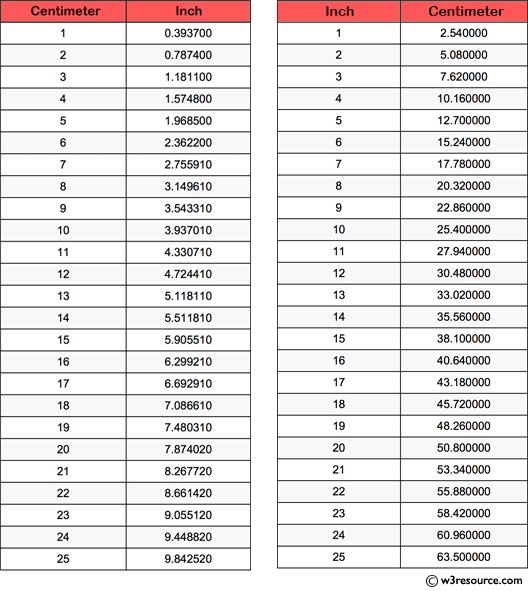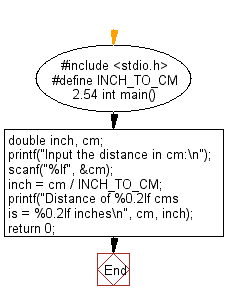﻿ C Program: Prints the corresponding value in inches - w3resource

# C Exercises: Prints the corresponding value in inches

## C Basic Declarations and Expressions: Exercise-54 with Solution

Write a C program that accepts a distance in centimeters and prints the corresponding value in inches.

Note: 1 inch = 2.54 cm.

Pictorial Presentation:Sample Solution:

C Code:

``````#include <stdio.h>
// Define a constant for converting inches to centimeters
#define INCH_TO_CM 2.54

int main() {
double inch, cm;

// Prompt user to input the distance in centimeters
printf("Input the distance in cm:\n");
scanf("%lf", &cm);

// Convert centimeters to inches
inch = cm / INCH_TO_CM;

// Display the converted distance
printf("Distance of %0.2lf cms is = %0.2lf inches\n", cm, inch);

return 0;
}
``````

Sample Output:

```Input Data: 500cms
Input the distance in cm:
Distance of 500.00 cms is = 196.85 inches
```

Flowchart:C programming Code Editor:

What is the difficulty level of this exercise?

Test your Programming skills with w3resource's quiz.

﻿